Trial ends in
JoVE Science Education
Organic Chemistry II

A subscription to JoVE is required to view this content.
You will only be able to see the first 20 seconds.

Polarimeter
Dean-Stark Trap
Polymerization

# Polarimeter

### Overview

Source: Vy M. Dong and Diane Le, Department of Chemistry, University of California, Irvine, CA

This experiment will demonstrate the use of a polarimeter, which is an instrument used to determine the optical rotation of a sample. Optical rotation is the degree to which a sample will rotate polarized light. Optically active samples will rotate the plane of light clockwise (dextrorotatory), designated as d or (+), or counterclockwise (levorotatory), designated as l or (−).

### Principles

The polarimeter is a quantitative method used to determine the optical rotation of a chiral molecule. A molecule is considered chiral if it is non-superimposable on its mirror image. More specifically, chiral molecules that are mirror images of one another are called enantiomers (Figure 2). Enantiomers have the same physical properties such as melting point, boiling point, and solubility; however, they differ in the degree to which they polarize light. A pure (R)-enantiomer of a compound will rotate light in an equal but opposite direction as its (S)-enantiomer. If a mixture of compounds is racemic, meaning it contains an equal mixture of (R)- and (S)-enantiomers, then its optical rotation will be zero. Thus, polarimetry is a way to characterize and distinguish the identity between a pair of enantiomers.

A polarimeter works by shining monochromatic light through a polarizer, which generates a beam of linearly polarized light. The polarized light will then rotate after it passes through a polarimetry cell containing the sample. An analyzer will then rotate counterclockwise or clockwise to allow the light to pass through and reach the detector (Figure 1). Using this instrument, the specific rotation of light can be calculated, which relates the observed optical rotation with the concentration of solution and cell pathlength. The specific rotation is defined by the following equation: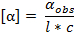where αobs is the observed optical rotation value given by the polarimeter, l is the cell pathlength in dm, and c is the concentration of the solution in g/mL.

Moreover, the enantiomeric excess (ee), which is a measurement of how much of one enantiomer exists over the other in a mixture, can be determined by using specific rotation. The calculation of ee is given by the following equation: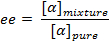where αmixture is the specific rotation of the mixture of enantiomers and αpure is the specific rotation of the pure enantiomer. Generally, if two out of three values in the equation are known (i.e., ee and αmixture) then the third value (αpure) can be calculated.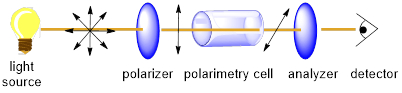Figure 1. Concept behind the polarimeter.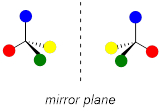Figure 2. Chiral molecules that are mirror images of one another are enantiomers.

### Procedure

1. Preparing the Polarimeter

1. Turn on instrument and let it warm up for 10 min.
2. Make sure instrument is set to "optical rotation" mode.
3. Prepare a blank sample in the polarimeter cell (1.5 mL total sample volume, 1 dm in length) containing only CHCl3. Make sure there are no air bubbles present.
4. Place the blank cell in the holder and press "zero."

2. Preparation of Analyte Sample

1. Prepare a stock solution of 10-15 mg of the chiral analyte in 1.5 mL CHCl3. Note the exact amount of compound used.

3. Measuring Optical Rotation

1. Fill the cell with 1.5 mL of the prepared stock solution containing the sample.
2. Place the cell in the holder and press "measure." The machine readout will give the optical rotation value. Remember to record the temperature as well.

4. Calculation of Specific Rotation

1. The specific rotation of a compound is defined by the following equation: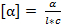where α is the optical rotation value given by the polarimeter, l is the cell pathlength in dm, and c is the concentration of the solution in g/mL.

Polarimeters are widely used in organic and analytical chemistry to assess the purity of a chemical product and investigate its properties.

Polarimeters detect the presence of enantiomers: mirror-image variants of a compound that may have wildly divergent biological activities. Distinguishing between enantiomers is critical in many applications, including pharmaceuticals, since one enantiomer is typically responsible for biological effects while the other is usually inert, less active, or, as in the case of the drug thalidomide, harmful.

This video will illustrate the principles of polarimetry, demonstrate setup and operation of a polarimeter, and discuss some applications.

Polarimetry is useful for studying organic compounds containing stereocenters.

Stereocenters are carbon atoms that are bonded to four different atoms or groups. In this example, the carbon atom is bonded to hydrogen, fluorine, chlorine, and bromine, forming bromo-chloro-fluoro-methane.

Compounds containing stereocenters are called "chiral," meaning they exist as mirror-image isomers: non-equivalent physical structures that cannot be rotated or oriented to superimpose on each other. The mirror-image isomers are called "enantiomers," and they have identical physical properties, with one exception related to optics.

In optics, non-laser light sources emit light waves that oscillate in a variety of planes. Such light waves are called "unpolarized." However, certain materials are capable of filtering light waves based on their plane of oscillation, transmitting only those light waves that oscillate in one specific plane while absorbing those oscillating in other planes. The transmitted light has been "plane polarized."

Enantiomers have different effects on plane polarized light. If they are struck by plane polarized light, one enantiomer will rotate the plane of oscillation clockwise, while the other will rotate the plane of oscillation by an equal angle counterclockwise. The former is called the "dextrorotatory" enantiomer, and its name prefixed with a plus sign. The latter is called the "levorotatory" enantiomer, and its name is prefixed with a minus sign. The ratio of rotation angle to concentration is unique for each compound, and is called "specific optical rotation."

A polarimeter detects whether one or both enantiomers are present in a sample. It consists of a light source, a polarizer, a sample cell, a detector, and an analyzer. The light source emits light waves that are unpolarized but monochromatic, meaning they have the same wavelength. The light waves then encounter the polarizer, which transmits only those oscillating in one specific plane, yielding a plane-polarized beam. The plane-polarized light then interacts with the sample in the sample cell.

If the sample contains only one enantiomer of the chiral compound, the polarized light will rotate. The angle is called the "optical rotation," and it depends on the specific optical rotation of the compound, its concentration, and the length of the sample cell. If, on the other hand, both enantiomers are present in equal concentrations, they form a "racemic mixture" that cannot rotate polarized light. Finally, if one enantiomer is present in greater concentration than the other, an "enantiomeric excess" results, and the plane of oscillation will be rotated in proportion to the excess.

After the polarized light passes through the sample, it is detected. The analyzer measures the optical rotation.

Now that you've seen the principles, let's examine a typical operating procedure.

The first step to using the polarimeter is zeroing the instrument.

First, turn on the polarimeter and let it warm up for 10 min.

Set the instrument to optical rotation mode.

The sample cell is typically a tube 1 dm long with a volume of 1.5 mL. Prepare the cell by cleaning with acetone and lab wipes.

Gently place the empty sample cell into the holder and press "zero." This establishes the baseline.

Next, calibrate the polarimeter using a pure sample of the chiral compound under investigation.

In this example, the dextrorotatory enantiomer of carvone is used. Pipette 1.5 mL into the sample cell. Insert the cell into the holder, and press "measure." The optical rotation is displayed. Dividing the measured optical rotation by concentration, or density for pure substances, and cell length yields the specific optical rotation of the compound.

The specific optical rotation of a purified unknown can be found similarly, by dissolving the unknown in an optically inactive solvent and measuring the optical rotation. The specific optical rotation of the compound is then determined by dividing by the concentration. The compound is then identified by comparing its specific optical rotation to literature values.

Now that you know how to perform measurements, we will explore some practical applications.

In the pharmaceutical industry, polarimetry is used for quality control. For instance, it has been used to measure the concentration and enantiomeric purity of ephedrine in commercial cough suppressants. Even in the presence of other ingredients, this technique can be used to determine the ephedrine concentration to within 1%.

In the food and beverage industries, sucrose concentrations and purities are monitored continuously with specially-designed flow polarimeters. Sucrose, one of the most common ingredients in foods, has a specific optical rotation of 66.5 degrees. By dividing the optical rotation of the sucrose stream by the specific optical rotation of sucrose, the concentration can be determined. Fluctuations in the optical rotation would indicate fluctuations in sucrose concentration.

Polarimetry has also been used to study reaction kinetics, including kinetics for enzyme systems such as the penicillin-penicillinase system. In this case, the sample cell contains both enzyme and substrate, and the optical rotation is measured with respect to time. The change in optical rotation is directly proportional to the change in substrate concentration. This not only reveals the reaction kinetics, but also permits simultaneous determination of enzyme and substrate concentrations in future assays.

You've just watched JoVE's introduction to the polarimeter. You should now understand its principles of operation, the steps for setup and measurement, and some of its applications. Thanks for watching!

### Results

Representative results for the measurement and calculation of specific rotation for Procedures 1-4.

 Procedure Step Reading on polarimeter 1.4 0.000 3.2 +0.563 4.1 [α]25D = +77° (c 0.73, CHCl3)

Table 1. Representative results for procedures 1-4.

### Applications and Summary

In this experiment, we have demonstrated the principles behind the polarimeter and how to measure and calculate the specific rotation of an optically active compound.

The polarimeter is an important instrument in the fine-chemical and pharmaceutical industries to assess the identity, purity, and quality of a compound. It is specifically used for the measurement of optical rotation of chiral compounds, which can be used to distinguish the identity of two enantiomers by confirming whether it is an (R) or (S) compound. This is especially important in pharmaceutical drug synthesis because one enantiomer is generally responsible for the biological effects while the other enantiomer is often less active and can have adverse effects. In addition, the polarimeter can be implemented to determine the unknown ee of a sample. If the ee value is unknown, this can be calculated using the polarimeter by determining the specific rotation.X